# Synchronous Machine

## Brushless DC Motor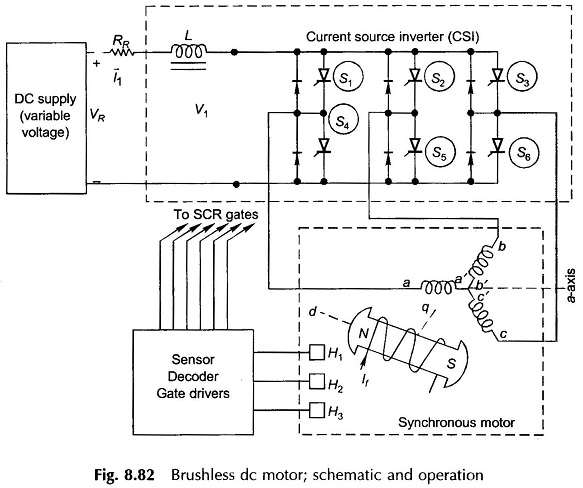Brushless DC Motor: The term Brushless DC Motor is applied to many configurations of ac synchronous motors in which semiconductor control is used to control stator currents such that maximum torque is obtained at a given speed. In a conventional motor the mechanical contactor, the commutator, maintains 90° elect degrees space displacement between the rotor …

## Single Phase Synchronous Generator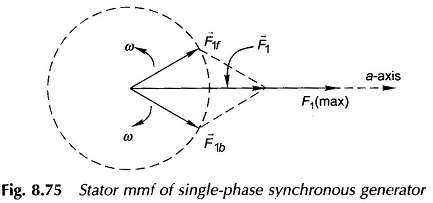Single Phase Synchronous Generator: Certain applications, usually restricted to less than 10 kVA are better served by a Single Phase Synchronous Generator. Examples are emergency, domestic/office supply, portable power for construction tools etc. Because of simplicity of distribution wiring, these loads are better served by a single-phase arrangement. Single Phase Synchronous Generator stator winding can …

## Short Circuit Current in Synchronous Generator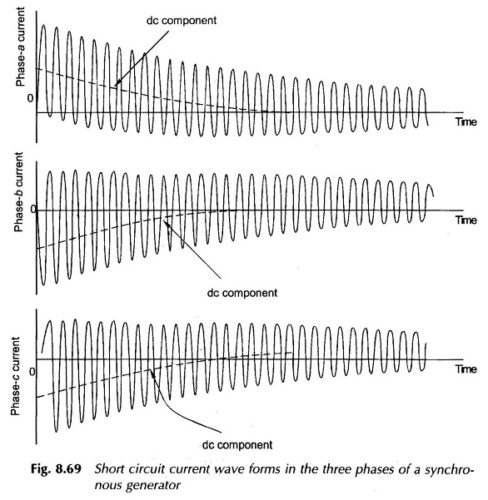Short Circuit Current in Synchronous Generator: The Short Circuit Current in Synchronous Generator being electrical in nature is much faster than the electromechanical dynamics discussed already. It will, therefore, be assumed that by the time the major features of the short-circuit phenomenon are over, the rotor speed remains constant at the synchronous value. The Short …

## Hunting in Synchronous Machine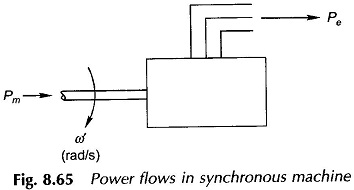Hunting in Synchronous Machine: So far the operation of the synchronous machine under steady-state conditions at constant (synchronous) speed has been considered. Under these conditions of Hunting in Synchronous Machine where Pe= electrical power output of the machine Pm= mechanical power input to the machine the machine losses having been assumed negligible. These power flows …

## Parallel Operation of Synchronous Generator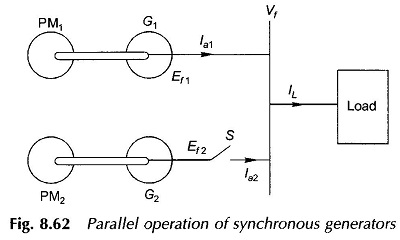Parallel Operation of Synchronous Generator: We already know that synchronous machine connected to infinite bus-bars. Parallel Operation of Synchronous Generator of two finite size will be considered, which is the way large practical size generators are used. In a power system the generators are connected to the nodes of a grid composed of a network …

## Slip Test on Synchronous Machine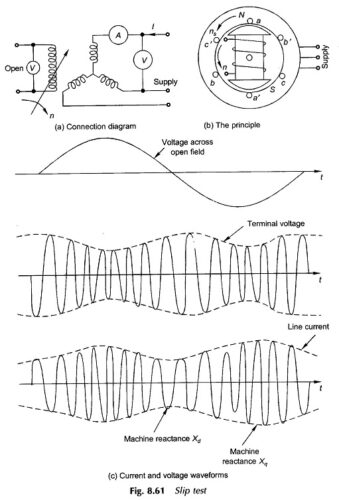Slip Test on Synchronous Machine: Direct and quadrature axis reactances of a salient-pole synchronous machine can be estimated by means of a test known as the Slip Test on Synchronous Machine. The machine armature is connected to a 3-phase supply whose voltage is much less than the rated voltage of the machine, while the rotor …

## Two Reaction Model of Salient Pole Synchronous Machine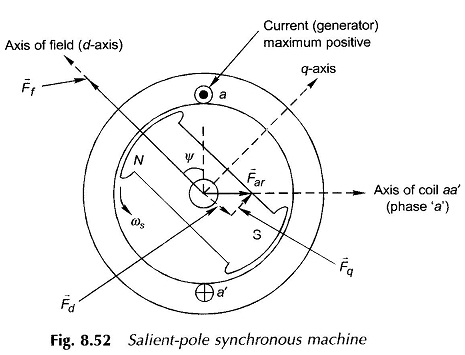Two Reaction Model of Salient Pole Synchronous Machine: In a Two Reaction Model of Salient Pole Synchronous Machine, the flux established by a mmf wave is independent of the spatial position of the wave axis with respect to the field pole axis. On the other hand, in a salient-pole machine as shown in the cross-sectional …

## Capability Curve of Synchronous Generator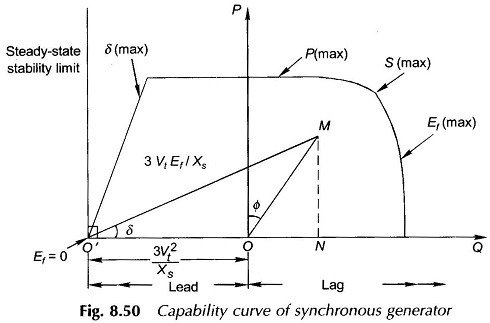Capability Curve of Synchronous Generator: Capability Curve of Synchronous Generator defines the bounds within which it can operate safely. Various bounds imposed on the machine are: MVA-loading cannot exceed the generator rating. This limit is imposed by the stator heating. MW-loading cannot exceed the turbine rating which is given by MVA (rat­ing) x pf (rating). …

## Power Flow Equation of Synchronous Generator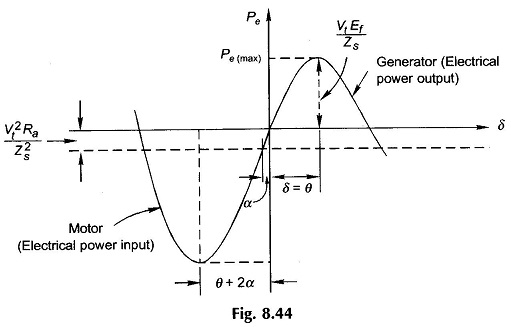Power Flow Equation of Synchronous Generator: Power Flow Equation of Synchronous Generator – The flow of active and reactive power in a synchronous link will now be studied. The approach will be analytical and armature resistance will be considered for generality of results. Figure 8.42 shows the schematic diagram of a synchronous generator wherein E̅f leads …

## Synchronous Machine Operation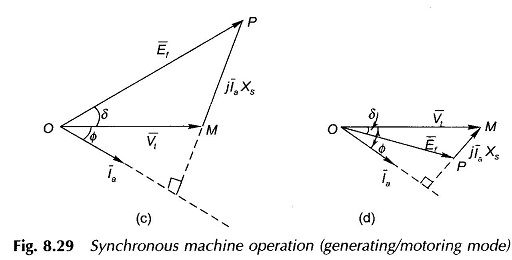Synchronous Machine Operation: The Synchronous Machine Operation are examined here under conditions of variable load and variable excitation. One of these quantities will be assumed to be held constant at a time while the other will be allowed to vary over a wide range. Further, here too the armature resistance will be assumed negligible. This …

Scroll to Top